### Learning Outcomes

• Isolate square roots in equations and solve for a variable
• Identify extraneous solutions to radical equations
• Square Roots and Completing the Square for Solving Radical Equations
• Use square roots to solve quadratic equations
• Complete the square to solve a quadratic equation
• Write a quadratic equation in standard form and identify the values of a, b, and c in a standard form quadratic equation.
• Use the Quadratic Formula to find all real solutions.
• Solve application problems requiring the use of the Quadratic Formula.

A basic strategy for solving radical equations is to isolate the radical term first, and then raise both sides of the equation to a power to remove the radical. (The reason for using powers will become clear in a moment.) This is the same type of strategy you used to solve other, non-radical equations—rearrange the expression to isolate the variable you want to know, and then solve the resulting equation.

The solutions of $x^2=a$ are called the square roots of a.

• When a is positive, a > 0, $x^2=a$ has two solutions, $+\sqrt{a},-\sqrt{a}$. $+\sqrt{a}$ is the nonnegative square root of a, and $-\sqrt{a}$ is the negative square root of a.
• When a is negative, a < 0, $x^2=a$ has no solutions.
• When a is zero, a = 0, $x^2=a$ has one solution: a = 0

Just to drive home the importance of the concept that when a is negative, a < 0, $x^2=a$ has no solutions, we will restate it in words.  If you have a negative number under a square root sign as in this example,

$\sqrt{-3}$

There will be no real number solutions.

There are two key ideas that you will be using to solve radical equations. The first is that if $a=b$, then ${{a}^{2}}={{b}^{2}}$. (This property allows you to square both sides of an equation and remain certain that the two sides are still equal.) The second is that if the square root of any nonnegative number x is squared, then you get x: ${{\left( \sqrt{x} \right)}^{2}}=x$. (This property allows you to “remove” the radicals from your equations.)

Let’s start with a radical equation that you can solve in a few steps:$\sqrt{x}-3=5$.

### Example

Solve. $\sqrt{x}-3=5$

To check your solution, you can substitute 64 in for x in the original equation. Does $\sqrt{64}-3=5$? Yes—the square root of 64 is 8, and $8−3=5$.

Notice how you combined like terms and then squared both sides of the equation in this problem. This is a standard method for removing a radical from an equation. It is important to isolate a radical on one side of the equation and simplify as much as possible before squaring. The fewer terms there are before squaring, the fewer additional terms will be generated by the process of squaring.

In the example above, only the variable x was underneath the radical. Sometimes you will need to solve an equation that contains multiple terms underneath a radical. Follow the same steps to solve these, but pay attention to a critical point—square both sides of an equation, not individual terms. Watch how the next two problems are solved.

### Example

Solve. $\sqrt{x+8}=3$

In the following video we show how to solve simple radical equations.

### Example

Solve. $1+\sqrt{2x+3}=6$

2. Square both sides of the equation: If $x=y$ then $x^{2}=y^{2}$.
3. Once the radical is removed, solve for the unknown.

## Identify Extraneous Solutions

Following rules is important, but so is paying attention to the math in front of you—especially when solving radical equations. Take a look at this next problem that demonstrates a potential pitfall of squaring both sides to remove the radical.

### Example

Solve. $\sqrt{a-5}=-2$

Look at that—the answer $a=9$ does not produce a true statement when substituted back into the original equation. What happened?

Check the original problem: $\sqrt{a-5}=-2$. Notice that the radical is set equal to $−2$, and recall that the principal square root of a number can only be positive. This means that no value for a will result in a radical expression whose positive square root is $−2$! You might have noticed that right away and concluded that there were no solutions for a.

Incorrect values of the variable, such as those that are introduced as a result of the squaring process are called extraneous solutions. Extraneous solutions may look like the real solution, but you can identify them because they will not create a true statement when substituted back into the original equation. This is one of the reasons why checking your work is so important—if you do not check your answers by substituting them back into the original equation, you may be introducing extraneous solutions into the problem.
In the next video example, we solve more radical equations that may have extraneous solutions.

Have a look at the following problem. Notice how the original problem is $x+4=\sqrt{x+10}$, but after both sides are squared, it becomes ${{x}^{2}}+8x+16=x+10$. Squaring both sides may have introduced an extraneous solution.

### Example

Solve. $x+4=\sqrt{x+10}$

### Example

Solve. $4+\sqrt{x+2}=x$

In the last video example we solve a radical equation with a binomial term on one side.

## Square Roots and Completing the Square

Quadratic equations can be solved in many ways. In the previous section we introduced the idea that solutions to radical equations in general can be found using these facts:

The solutions of $x^2=a$ are called the square roots of a.

• When a is positive, a > 0, $x^2=a$ has two solutions, $+\sqrt{a},-\sqrt{a}$. $+\sqrt{a}$ is the nonnegative square root of a, and $-\sqrt{a}$ is the negative square root of a.
• When a is negative, a < 0, $x^2=a$ has no solutions.
• When a is zero, a = 0, $x^2=a$ has one solution: a = 0

A shortcut way to write $\sqrt{a}$ or $-\sqrt{a}$ is $\pm \sqrt{a}$. The symbol $\pm$ is often read “positive or negative.” If it is used as an operation (addition or subtraction), it is read “plus or minus.”

### Example

Solve using the Square Root Property. $x^{2}=9$

Notice that there is a difference here in solving $x^{2}=9$ and finding $\sqrt{9}$. For $x^{2}=9$, you are looking for all numbers whose square is 9. For $\sqrt{9}$, you only want the principal (nonnegative) square root. The negative of the principal square root is $-\sqrt{9}$; both would be $\pm \sqrt{9}$. Unless there is a symbol in front of the radical sign, only the nonnegative value is wanted!

In the example above, you can take the square root of both sides easily because there is only one term on each side. In some equations, you may need to do some work to get the equation in this form. You will find that this involves isolating $x^{2}$.

### Example

Solve. $10x^{2}+5=85$

Sometimes more than just the x is being squared:

### Example

Solve. $\left(x–2\right)^{2}–50=0$

In this video example, you will see more examples of solving quadratic equations using square roots.

### Completing the Square to Solve a Quadratic Equation

Of course, quadratic equations often will not come in the format of the examples above. Most of them will have x terms. However, you may be able to factor the expression into a squared binomial—and if not, you can still use squared binomials to help you.

Some of the above examples have squared binomials: $\left(1+r\right)^{2}$ and $\left(x–2\right)^{2}$ are squared binomials. If you expand these, you get a perfect square trinomial.

Perfect square trinomials have the form $x^{2}+2xs+s^{2}$ and can be factored as $\left(x+s\right)^{2}$, or they have the form $x^{2}–2xs+s^{2}$ and can be factored as $\left(x–s\right)^{2}$. Let’s factor a perfect square trinomial into a squared binomial.

### Example

Factor $9x^{2}–24x+16$.

You can use the procedure in this next example to help you solve equations where you identify perfect square trinomials, even if the equation is not set equal to 0.

### Example

Solve. $4x^{2}+20x+25=8$

One way to solve quadratic equations is by completing the square. When you don’t have a perfect square trinomial, you can create one by adding a constant term that is a perfect square to both sides of the equation. Let’s see how to find that constant term.

“Completing the square” does exactly what it says—it takes something that is not a square and makes it one. This idea can be illustrated using an area model of the binomial $x^{2}+bx$.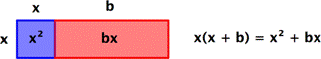In this example, the area of the overall rectangle is given by $x\left(x+b\right)$.

Now let’s make this rectangle into a square. First, divide the red rectangle with area bx into two equal rectangles each with area $\frac{b}{2}x$. Then rotate and reposition one of them. You haven’t changed the size of the red area—it still adds up to $bx$.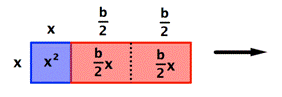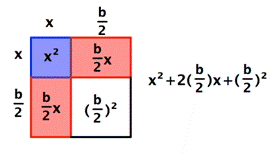The red rectangles now make up two sides of a square, shown in white. The area of that square is the length of the red rectangles squared, or ${{\left( \frac{b}{2} \right)}^{2}}$.

Here comes the cool part—do you see that when the white square is added to the blue and red regions, the whole shape is also now a square? In other words, you’ve “completed the square!” By adding the quantity $\left(\frac{b}{2}\right)^{2}$ to the original binomial, you’ve made a square, a square with sides of $x+\frac{b}{2}$.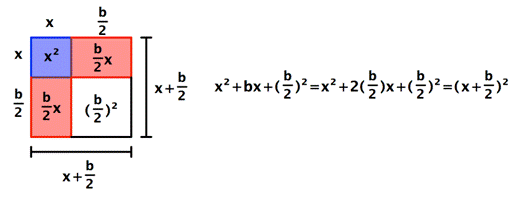Notice that the area of this square can be written as a squared binomial: $\left(x+\frac{b}{2}\right)^{2}$.

### Finding a Value that will Complete the Square in an Expression

To complete the square for an expression of the form $x^{2}+bx$:

• Identify the value of b;
• Calculate and add $\left(\frac{b}{2}\right)^{2}$.

The expression becomes $x^{2}+bx+\left(\frac{b}{2}\right)^{2}=\left(x+\frac{b}{2}\right)^{2}$.

### Example

Find the number to add to $x^{2}+8x$ to make it a perfect square trinomial.

Notice that ${{\left( \frac{b}{2} \right)}^{2}}$ is always positive, since it is the square of a number. When you complete the square, you are always adding a positive value.

In the following video, we show more examples of how to find a constant terms that will make a trinomial a perfect square.

You can use completing the square to help you solve a quadratic equation that cannot be solved by factoring.

Let’s start by seeing what happens when you complete the square in an equation. In the example below, notice that completing the square will result in adding a number to both sides of the equation—you have to do this in order to keep both sides equal!

### Example

Rewrite $x^{2}+6x=8$ so that the left side is a perfect square trinomial.

Can you see that completing the square in an equation is very similar to completing the square in an expression? The main difference is that you have to add the new number ($+9$ in this case) to both sides of the equation to maintain equality.

Now let’s look at an example where you are using completing the square to actually solve an equation, finding a value for the variable.

### Example

Solve. $x^{2}–12x–4=0$

In this last video, we solve more quadratic equations by completing the square.

You may have noticed that because you have to use both square roots, all the examples have two solutions. Here is another example that’s slightly different.

### Example

Solve $x^{2}+16x+17=-47$.

Take a closer look at this problem and you may see something familiar. Instead of completing the square, try adding 47 to both sides in the equation. The equation $x^{2}+16x+17=−47$ becomes $x^{2}+16x+64=0$. Can you factor this equation using grouping? (Think of two numbers whose product is 64 and whose sum is 16).

It can be factored as $(x+8)(x+8)=0$, of course! Knowing how to complete the square is very helpful, but it is not always the only way to solve an equation.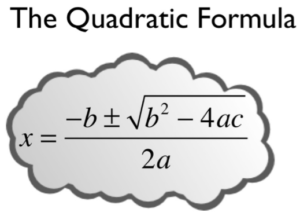You can solve any quadratic equation by completing the square—rewriting part of the equation as a perfect square trinomial. If you complete the square on the generic equation $ax^{2}+bx+c=0$ and then solve for x, you find that $x=\frac{-b\pm \sqrt{{{b}^{2}}-4ac}}{2a}$. This equation is known as the Quadratic Formula.

This formula is very helpful for solving quadratic equations that are difficult or impossible to factor, and using it can be faster than completing the square. The Quadratic Formula can be used to solve any quadratic equation of the form $ax^{2}+bx+c=0$.

The form $ax^{2}+bx+c=0$ is called standard form of a quadratic equation. Before solving a quadratic equation using the Quadratic Formula, it’s vital that you be sure the equation is in this form. If you don’t, you might use the wrong values for a, b, or c, and then the formula will give incorrect solutions.

### Example

Rewrite the equation $3x+2x^{2}+4=5$ in standard form and identify a, b, and c.

### Example

Rewrite the equation $2(x+3)^{2}–5x=6$ in standard form and identify a, b, and c.

The Quadratic Formula will work with any quadratic equation, but only if the equation is in standard form, $ax^{2}+bx+c=0$. To use it, follow these steps.

• Put the equation in standard form first.
• Identify the coefficients, a, b, and c. Be careful to include negative signs if the bx or c terms are subtracted.
• Substitute the values for the coefficients into the Quadratic Formula.
• Simplify as much as possible.
• Use the $\pm$ in front of the radical to separate the solution into two values: one in which the square root is added, and one in which it is subtracted.
• Simplify both values to get the possible solutions.

That’s a lot of steps. Let’s try using the Quadratic Formula to solve a relatively simple equation first; then you’ll go back and solve it again using another factoring method.

### Example

Use the Quadratic Formula to solve the equation $x^{2}+4x=5$.

You can check these solutions by substituting $1$ and $−5$ into the original equation.

 $\begin{array}{r}x=1\\x^{2}+4x=5\\\left(1\right)^{2}+4\left(1\right)=5\\1+4=5\\5=5\end{array}$ $\begin{array}{r}x=-5\\x^{2}+4x=5\,\,\,\,\,\\\left(-5\right)^{2}+4\left(-5\right)=5\,\,\,\,\,\\25-20=5\,\,\,\,\,\\5=5\,\,\,\,\,\end{array}$

You get two true statements, so you know that both solutions work: $x=1$ or $-5$. You’ve solved the equation successfully using the Quadratic Formula!

The power of the Quadratic Formula is that it can be used to solve any quadratic equation, even those where finding number combinations will not work.

In teh following video, we show an example of using the quadratic formula to solve an equation with two real solutions.

Most of the quadratic equations you’ve looked at have two solutions, like the one above. The following example is a little different.

### Example

Use the Quadratic Formula to solve the equation $x^{2}-2x=6x-16$.

Again, check using the original equation.

$\begin{array}{r}x^{2}-2x=6x-16\,\,\,\,\,\\\left(4\right)^{2}-2\left(4\right)=6\left(4\right)-16\\16-8=24-16\,\,\,\,\,\,\\8=8\,\,\,\,\,\,\,\,\,\,\,\,\,\,\,\,\,\,\,\,\,\,\end{array}$

In the following video we show an example of using the quadratic formula to solve a quadratic equation that has one repeated solution.

In this video example we show that solutions to quadratic equations can have rational answers.

Quadratic equations are widely used in science, business, and engineering. Quadratic equations are commonly used in situations where two things are multiplied together and they both depend on the same variable. For example, when working with area, if both dimensions are written in terms of the same variable, you use a quadratic equation. Because the quantity of a product sold often depends on the price, you sometimes use a quadratic equation to represent revenue as a product of the price and the quantity sold. Quadratic equations are also used when gravity is involved, such as the path of a ball or the shape of cables in a suspension bridge.

A very common and easy-to-understand application is the height of a ball thrown at the ground off a building. Because gravity will make the ball speed up as it falls, a quadratic equation can be used to estimate its height any time before it hits the ground. Note: The equation isn’t completely accurate, because friction from the air will slow the ball down a little. For our purposes, this is close enough.

### Example

A ball is thrown off a building from 200 feet above the ground. Its starting velocity (also called initial velocity) is $−10$ feet per second. (The negative value means it’s heading toward the ground.)

The equation $h=-16t^{2}-10t+200$ can be used to model the height of the ball after t seconds. About how long does it take for the ball to hit the ground?

The area problem below does not look like it includes a Quadratic Formula of any type, and the problem seems to be something you have solved many times before by simply multiplying. But in order to solve it, you will need to use a quadratic equation.

### Example

Bob made a quilt that is 4 ft $\times$ 5 ft. He has 10 sq. ft. of fabric he can use to add a border around the quilt. How wide should he make the border to use all the fabric? (The border must be the same width on all four sides.)

In this last video, we show how to use the quadratic formula to solve an application involving a picture frame.

## Summary

A common method for solving radical equations is to raise both sides of an equation to whatever power will eliminate the radical sign from the equation. But be careful—when both sides of an equation are raised to an even power, the possibility exists that extraneous solutions will be introduced. When solving a radical equation, it is important to always check your answer by substituting the value back into the original equation. If you get a true statement, then that value is a solution; if you get a false statement, then that value is not a solution.

Completing the square is used to change a binomial of the form $x^{2}+bx$ into a perfect square trinomial ${{x}^{2}}+bx+{{\left( \frac{b}{2} \right)}^{2}}$, which can be factored to ${{\left( x+\frac{b}{2} \right)}^{2}}$. When solving quadratic equations by completing the square, be careful to add ${{\left( \frac{b}{2} \right)}^{2}}$ to both sides of the equation to maintain equality. The Square Root Property can then be used to solve for x. With the Square Root Property, be careful to include both the principal square root and its opposite. Be sure to simplify as needed.

Quadratic equations can appear in different applications. The Quadratic Formula is a useful way to solve these equations, or any other quadratic equation! The Quadratic Formula, $x=\frac{-b\pm \sqrt{{{b}^{2}}-4ac}}{2a}$, is found by completing the square of the quadratic equation .